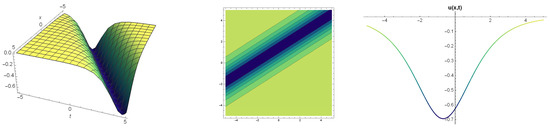Next Article in Journal
Models of Set Theory in which Nonconstructible Reals First Appear at a Given Projective Level
Next Article in Special Issue
Establishing New Criteria for Oscillation of Odd-Order Nonlinear Differential Equations
Previous Article in Journal
Effect of a Boundary Layer on Cavity Flow
Previous Article in Special Issue
Improved Oscillation Criteria for 2nd-Order Neutral Differential Equations with Distributed Deviating Arguments
Article

# Construction of Different Types Analytic Solutions for the Zhiber-Shabat Equation

1
Department of Actuary, Faculty of Science, Firat University, Elazig 23200, Turkey
2
Department of Computer Engineering, Faculty of Engineering, Ardahan University, Ardahan 75000, Turkey
3
Department of Basic Sciences, University of Engineering and Technology, Peshawar 25000, Pakistan
4
School of Mathematics and Information Science, Henan Polytechnic University, Jiaozuo 454000, China
*
Author to whom correspondence should be addressed.
These authors contributed equally to this work.
Mathematics 2020, 8(6), 908; https://doi.org/10.3390/math8060908
Received: 10 May 2020 / Revised: 1 June 2020 / Accepted: 2 June 2020 / Published: 3 June 2020
In this paper, a new solution process of $( 1 / G ′ )$ -expansion and $( G ′ / G , 1 / G )$ -expansion methods has been proposed for the analytic solution of the Zhiber-Shabat (Z-S) equation. Rather than the classical $( G ′ / G , 1 / G )$ -expansion method, a solution function in different formats has been produced with the help of the proposed process. New complex rational, hyperbolic, rational and trigonometric types solutions of the Z-S equation have been constructed. By giving arbitrary values to the constants in the obtained solutions, it can help to add physical meaning to the traveling wave solutions, whereas traveling wave has an important place in applied sciences and illuminates many physical phenomena. 3D, 2D and contour graphs are displayed to show the stationary wave or the state of the wave at any moment with the values given to these constants. Conditions that guarantee the existence of traveling wave solutions are given. Comparison of $( G ′ / G , 1 / G )$ -expansion method and $( 1 / G ′ )$ -expansion method, which are important instruments in the analytical solution, has been made. In addition, the advantages and disadvantages of these two methods have been discussed. These methods are reliable and efficient methods to obtain analytic solutions of nonlinear evolution equations (NLEEs). View Full-Text
Show FiguresFigure 1

MDPI and ACS Style

Yokus, A.; Durur, H.; Ahmad, H.; Yao, S.-W. Construction of Different Types Analytic Solutions for the Zhiber-Shabat Equation. Mathematics 2020, 8, 908. https://doi.org/10.3390/math8060908

AMA Style

Yokus A, Durur H, Ahmad H, Yao S-W. Construction of Different Types Analytic Solutions for the Zhiber-Shabat Equation. Mathematics. 2020; 8(6):908. https://doi.org/10.3390/math8060908

Chicago/Turabian Style

Yokus, Asıf, Hülya Durur, Hijaz Ahmad, and Shao-Wen Yao. 2020. "Construction of Different Types Analytic Solutions for the Zhiber-Shabat Equation" Mathematics 8, no. 6: 908. https://doi.org/10.3390/math8060908

Find Other Styles
Note that from the first issue of 2016, MDPI journals use article numbers instead of page numbers. See further details here.

1